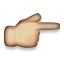LINQ method syntax challenge 2 help

I do not know what property name I am supposed to pass into this argument. In the video we had colors and names. In this there is the list of numbers. Can someone help explain what I'm missing in my code?

I have tried the following lines of code. But I do not know what I'm missing.

return _numbers.OrderByDescending((n) => n.NumbersGreaterThanFive);
return _numbers.OrderByDescending((n) => n.numbersGreaterThanFive);
return _numbers.OrderByDescending((n) => n.List);
return _numbers.OrderByDescending((n) => n._numbers);
NumberAnalysis.cs
using System.Collections.Generic;
using System.Linq;

namespace Treehouse.CodeChallenges
{
public class NumberAnalysis
{
private List<int> _numbers;
public NumberAnalysis()
{
_numbers = new List<int> { 2, 4, 6, 8, 10 };
}

public IEnumerable<int> NumbersGreaterThanFive()
{
return _numbers.Where((n) => n > 5);
}

public IEnumerable<int> ReverseNumbers()
{
return _numbers.OrderByDescending((n) => n.);
}
}
}Your lambda expression has a stray period (.) at the end.Also, while it does not cause a problem, you don't need parentheses around a single parameter in a lambda expression.